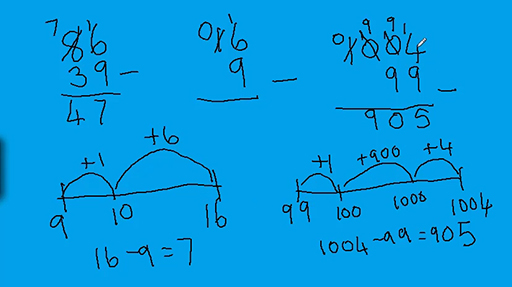Teaching mathematics

Start this free course now. Just create an account and sign in. Enrol and complete the course for a free statement of participation or digital badge if available.

Free course

# 3.2 Formal written methods for subtraction

Subtraction is experienced as being more difficult than addition. Subtraction is the inverse of addition and knowing this can help learners to use complementary addition in informal methods of subtraction.

Video 4 demonstrates the decomposition method (or column method) of subtraction and the empty number line method which uses complementary addition. This latter method is a visible example instantiation of the method which many people use mentally.

Download this video clip.Video player: Video 4 Choosing the best method for subtractionSkip transcript: Video 4 Choosing the best method for subtraction

#### Transcript: Video 4 Choosing the best method for subtraction

INSTRUCTOR:
So a lot of learners in school are taught to subtract in columns. So for example, if we've got 86 and I want to subtract 39, those would be put in columns, so the units are underneath each other, the tens underneath each other. And I'm going to subtract. Now, I can't do 6 take away 9. So I'm going to go over to the 8, that's eight 10s. I'm going to take one away, which will leave me with 7.
And then that 10 over here becomes 10 units. So together that makes 16 units, take away 9. 16 take 9 is 7. And then 7 take 3 is 4. So that seems quite straightforward. That's known as the decomposition method because we decompose the 8 into 7 tens and then 10 units over here.
This can sometimes be quite problematic. Sometimes, learners can just simply do the 9 take 6 and provide me with 3. And then 8 take 3 would be 5. So that's the very common mistake. They know they've got to subtract something so they just take the smaller from the larger and don't take any notice of whether the number's actually on the top or not.
Consider this. If I've got 16 take away 9 and I tried to do that using composition composition, I can't do 6 take 9. So I come here, take a 10 away, that leaves me with none. I put the 10 there is 10 units. That's now 16 take 9. And actually, I've got the same problem I had before, haven't I? So 16 take 9 doesn't really make terribly much sense using the decomposition method.
Or again, if I had a number like this 1,004 and supposing I wanted to subtract 99 and I wanted to use the decomposition method, I can't do 4 take 9. So I go to that, but there aren't any n or to there. So I've got to go all the way to the thousands. I'll take that 1,000 off and leaves with 0. Then I can put that there as 10 hundreds. I can now take 1 away from there and leave it with 9.
I've now got 10 tens. And I'll take one of those off and leave me with 9. And then I've got that 10 units. finally there. 14 take nine is 5. 9 take 9 is nothing. 9 take nothing is 9. And there isn't anything left in the thousands. Now I've done that accurately, but it is very messy. And it's fraught with difficulties, because you've got to go all the way to the thousands to decompose and put the numbers across.
So in the both of these cases, it would actually be better to think about those in a different way. Certainly, if we think of the number line, which was demonstrated in the previous section-- so if I was thinking about nine and what I need to add to make 16 I could say to myself, well to get to 10, I've added 1. And then 10 to 16, I've added 6. So that's 7. 16 take 9 is 7.
And over here, I could also use a number line. So if I were to decide what I need to add to 99 to make 1,004, so going from 99 to the next 100, I've added 1. 100 to 1,000, I've added 900. I should set out 1 there. And finally, that last little bit I added 4. So altogether, I've added 900, 4, and 1. So I've added 905. So 1,004 take 99 is 905.
Both of these subtractions made much better sense to do using a number line. Or you might think for this one, 99 is quite close to 100, so take 100 off and then add 1 back on. So 1,004 take off 100, 904, add 1 back on 905. So what it shows is that it rather depends on the problem you're trying to solve as to which is the best method to use.
End transcript: Video 4 Choosing the best method for subtraction
Video 4 Choosing the best method for subtraction
Interactive feature not available in single page view (see it in standard view).

## Activity 8 Choosing the most appropriate method of subtraction

Timing: Allow 8 minutes

Consider the following subtractions and decide whether it is more appropriate to use the decomposition method or the empty number line method.

• a.

• b.

• c.The length of a journey which starts at 9.55 a.m. and ends at 11.05 a.m.

### Discussion

If the numbers in the subtraction are close together then it makes more sense to ‘count up’ from the smaller to the larger, the method known as known as complementary addition. This is especially useful if the numbers bridge over a 10s or 100s sort of number or over the hour in a time calculation.

TM_1